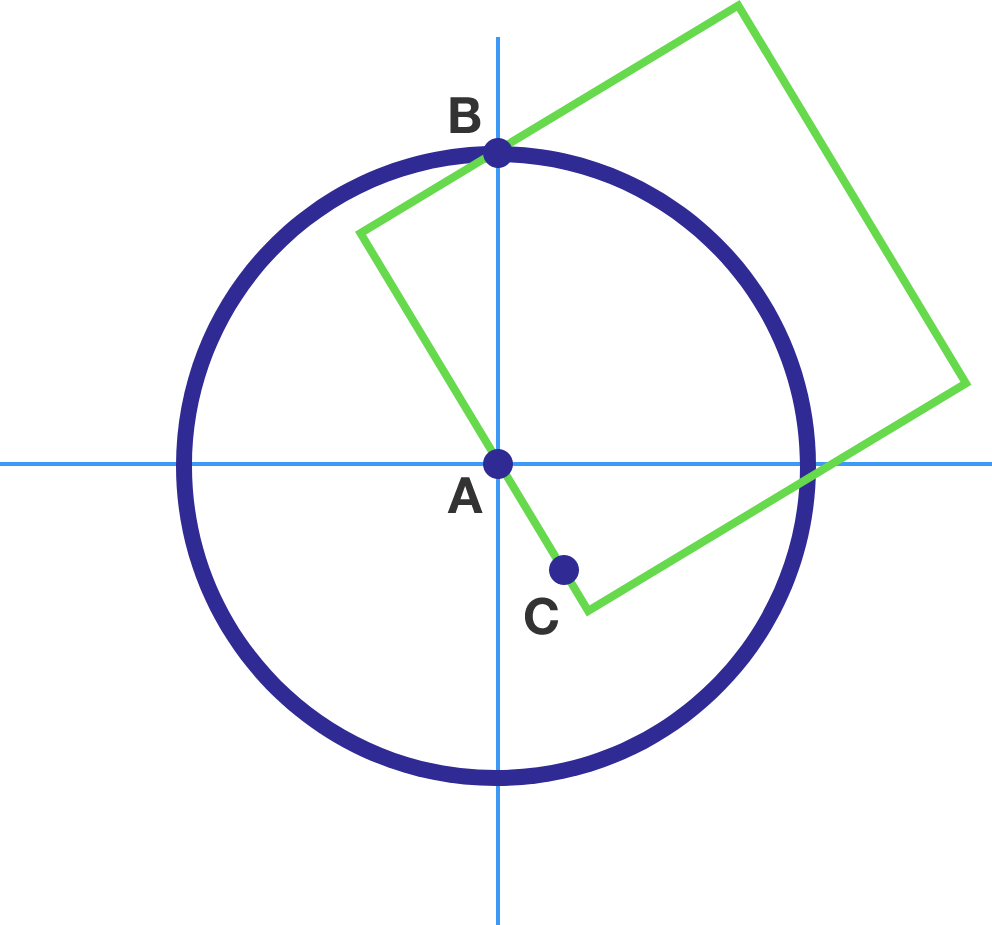# 3 points in a circle

Geometry Level 4

Consider two points: $A=(0,0)$ which is the center of a unit circle, and $B=(0,1)$ which lies on that unit circle. Now, you choose a third point $C$ inside the circle uniformly at random.What is the probability that you will be able to draw a square such that all three points $A, B, C$ lie on two adjacent sides of the square?